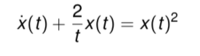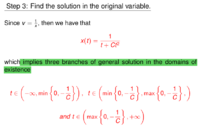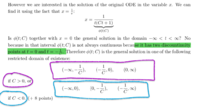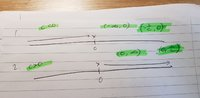# Finding restriction in differential equation

#### Probability 0.99

##### New memberI have managed to solve this Bernoulli equation and found the general solution for it using integrating factor but I am struggling to understand how the domains are derived. I get that x cannot be 0 because 2/0= 0 However why are there 3 branches? But why are there 3 branches? And what does the symbol E denote? I don't major in Maths, so I find this a bit hard to understand. Thanks

#### MarkFL

##### Super Moderator
Staff member
Yes, upon solving the given ODE, I get:

$$\displaystyle x(t)=\frac{1}{t(c_1t+1)}$$

Now, if we analyze the denominator of the solution, we find it has two zeroes:

$$\displaystyle t\in\left\{-\frac{1}{c_1},0\right\}$$

These two values will divide the real number line into 3 intervals, but since we cannot give the sign of the parameter, we must use the min and max functions as stated in your screenshot.

#### Probability 0.99

##### New member
Yes, upon solving the given ODE, I get:

$$\displaystyle x(t)=\frac{1}{t(c_1t+1)}$$

Now, if we analyze the denominator of the solution, we find it has two zeroes:

$$\displaystyle t\in\left\{-\frac{1}{c_1},0\right\}$$

These two values will divide the real number line into 3 intervals, but since we cannot give the sign of the parameter, we must use the min and max functions as stated in your screenshot.
Thanks for the help. I don't quite get the mathematical notation you used here; does it mean t is contained within the interval of x =-1/c1 and y=0?
I am a little confused as y is not involved in the differential equation...

#### MarkFL

##### Super Moderator
Staff member
The statement:

$$\displaystyle t\in\left\{-\frac{1}{c_1},0\right\}$$

means that $$t$$ is an element of the given set. Those are the two values of $$t$$ for which the denominator is zero.

$$\displaystyle y$$ isn't involved because $$t$$ is the independent variable, while $$x$$ is the dependent variable.

#### Probability 0.99

##### New member
The statement:

$$\displaystyle t\in\left\{-\frac{1}{c_1},0\right\}$$

means that $$t$$ is an element of the given set. Those are the two values of $$t$$ for which the denominator is zero.

$$\displaystyle y$$ isn't involved because $$t$$ is the independent variable, while $$x$$ is the dependent variable.
I really appreciate the help since I have been struggling to understand this for a while now. I have found further explanation for this question in my lecture notes.I can understand the max and min answer but making assumptions on whether C>0 or <0 really confuse me.
C>0 can I understand this as max {-1/c,0) and C<0 as the min {-1/c,0}. How exactly are the 3 domains for each condition deduced?
I apologise for the endless questions; this is hard to find help on this. Many thanks.

#### MarkFL

##### Super Moderator
Staff member
Okay, if we have 2 values of $$t$$ where the function is not defined, then that divides the domain of the function into 3 intervals...are you with me on that?

#### Probability 0.99

##### New member
Okay, if we have 2 values of $$t$$ where the function is not defined, then that divides the domain of the function into 3 intervals...are you with me on that?
Yes, I understand this part from the min and max explanation before. But after introducing C>0 and C<0, I no longer follow...

#### MarkFL

##### Super Moderator
Staff member
Yes, I understand this part from the min and max explanation before. But after introducing C>0 and C<0, I no longer follow...
Okay, now if $$C<0$$ then $$\displaystyle \frac{1}{C}<0$$, right?

#### Probability 0.99

##### New member
Okay, now if $$C<0$$ then $$\displaystyle \frac{1}{C}<0$$, right?
Yes, this means 1/c will have to be a negative number; I understand this.

C>0 means 1/C>0. I also get this part.

Last edited:

#### MarkFL

##### Super Moderator
Staff member
Yes, this means 1/c will have to be a negative number; I understand this.
Okay, good. So, when we're deciding the intervals, since $$t=0$$ is already outside of the domain of the function, it matters whether the other value outside of the domain is less than or greater than zero. Do you see why?

#### Probability 0.99

##### New member
Okay, good. So, when we're deciding the intervals, since $$t=0$$ is already outside of the domain of the function, it matters whether the other value outside of the domain is less than or greater than zero. Do you see why?
Is it because t=0 is the discontinuity point; hence this cannot be in our interval. Whereas any other values that are not 0 have the possibilities of being in that interval that is continuous?
I kind of get this part; where can we go from here? Thanks a lot.

#### MarkFL

##### Super Moderator
Staff member
We have two discontinuities in our domain:

$$\displaystyle t=0$$

and

$$\displaystyle t=-\frac{1}{C}$$

Now, if $$C<0$$ then $$\displaystyle -\frac{1}{C}>0$$

and our intervals would be:

$$\displaystyle (-\infty,0)$$

$$\displaystyle \left(0,-\frac{1}{C}\right)$$

$$\displaystyle \left(-\frac{1}{C},\infty\right)$$

But, if $$0<C$$ then $$\displaystyle -\frac{1}{C}<0$$

and our intervals would be:

$$\displaystyle \left(-\infty,-\frac{1}{C}\right)$$

$$\displaystyle \left(-\frac{1}{C},0\right)$$

$$\displaystyle \left(0,\infty\right)$$

Does that make sense?

#### Probability 0.99

##### New member
(-infinity ,0). I know this is the interval. But if you are to interpret this , how do you do so? Do you say t is in between -ininifty and 0?
And another another possibility is that t is between 0 and -1/c? Thank you

#### Probability 0.99

##### New member
We have two discontinuities in our domain:

$$\displaystyle t=0$$

and

$$\displaystyle t=-\frac{1}{C}$$

Now, if $$C<0$$ then $$\displaystyle -\frac{1}{C}>0$$

and our intervals would be:

$$\displaystyle (-\infty,0)$$

$$\displaystyle \left(0,-\frac{1}{C}\right)$$

$$\displaystyle \left(-\frac{1}{C},\infty\right)$$

But, if $$0<C$$ then $$\displaystyle -\frac{1}{C}<0$$

and our intervals would be:

$$\displaystyle \left(-\infty,-\frac{1}{C}\right)$$

$$\displaystyle \left(-\frac{1}{C},0\right)$$

$$\displaystyle \left(0,\infty\right)$$

Does that make sense?
(-infinity ,0). I know this is the interval. But if you are to interpret this , how do you do so? Do you say t is in between -ininifty and 0?
And another another possibility is that t is between 0 and -1/c? Thanks

#### MarkFL

##### Super Moderator
Staff member
Picture a real number line. Mark a point at zero. Now picture two identical number lines, both with the point at zero marked off. On one, mark another point to the left of zero, and on the other mark a point to the right of zero. Do you see how the 3 resulting intervals differ on the two number lines?

#### Probability 0.99

##### New member
Picture a real number line. Mark a point at zero. Now picture two identical number lines, both with the point at zero marked off. On one, mark another point to the left of zero, and on the other mark a point to the right of zero. Do you see how the 3 resulting intervals differ on the two number lines?I have taken your suggestion and came up with the following. For C<0, why did you put (0,-1/c) instead of (-1/C,0)? I thought that you must put the negative value on the left and the more positive one on the right...

If 0 is marked off because it cannot be included in the domain then how are (0,-1/C) in C<0 and (-1/C,0) deduced?

Thanks for the assistance!